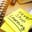Related Tags

javascript
comparison
operator
communitycreator

# How multiple comparison operators work in JavaScriptNon Stop Learning...

In JavaScript, automatic type conversion occurs as needed. Comparison operators take two numerical operands. If one of the operands is not of numerical type, it gets converted to a numerical before applying the operation.

Type conversion doesn’t work for all operators.

## Syntax

x < y < z


### Example 1

5 < 10 < 15

Let’s take the values above and see what happens.

• In this comparison, (x < y < z) is equivalent to ((x<y)<z).
• As both operators are <, they have the same precedence. However, since < has left associativity, the expression is evaluated from left to right. Therefore, 5 < 10 is executed first, and returns true.
• Next to be executed is true < 15. As discussed earlier, type conversion happens, and true is converted to 1.
• The effective expression is now 1 < 15, which returns true.

### Example 2

15 > 10 > 5

Let’s take the values above and see what happens.

• In this comparison, (x > y > z) is equivalent to ((x>y)>z).
• As both operators are >, they have the same precedence. However, since > has left associativity, the expression is evaluated from left to right. Therefore, 15 > 10 is executed first and returns true.
• Next to be executed is true > 5. As discussed earlier, type conversion happens and true is converted to 1.
• The effective expression is now 1 > 5, which returns false.

## Code

// Example 1
x = 5 < 10
console.log("5 < 10 is " + x)
console.log(x + " < 15 is " + (x < 15))

// Example 2
y = 15 > 10
console.log("15 > 10 is " + y)
console.log(y + " > 5 is " + (y > 5))

## Conclusion

These cases can also be solved using logical operators.

Multiple comparison operators are more complex to understand and do not improve the readability of the code. On the other hand, logical operators (AND, OR, NOT) provide more readability and are easy to understand.

Therefore, multiple comparison operators should be avoided by using the AND and OR operators to split the expression.

RELATED TAGS

javascript
comparison
operator
communitycreator

CONTRIBUTORNon Stop Learning...
RELATED COURSES

View all Courses

Keep Exploring

Learn in-demand tech skills in half the time